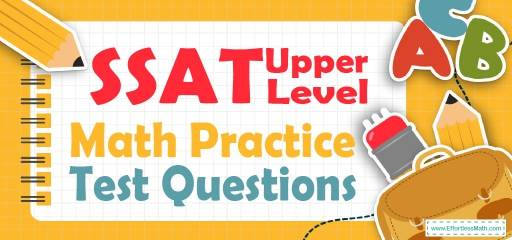# SSAT Upper Level Math Practice Test QuestionsPreparing your students for the SSAT Upper-Level Math test? Try these free SSAT Upper-Level Math Practice questions. Reviewing practice questions is the best way to brush up on your student’s Math skills. Here, we walk you through solving 10 common SSAT Upper-Level Math practice problems covering the most important math concepts on the SSAT Upper-Level Math test.

These SSAT Upper-Level Math practice questions are designed to be similar to those found on the real SSAT Upper-Level Math test. They will assess your student’s level of preparation and will give you a better idea of what students need to study on their exams.

## 10 Sample SSAT Upper Level Math Practice Questions

1- The sum of six different negative integers is $$-80$$. If the smallest of these integers is $$-16$$, what is the largest possible value of one of the other five integers?

A. $$-16$$

B. $$-10$$

C. $$-8$$

D. $$-4$$

E. $$-1$$

2- What is the slope of a line that is perpendicular to the line $$2x-4y=24$$?

A. $$-2$$

B. $$-\frac{1}{2}$$

C. 6

D. 12

E. 14

3- The width of a box is one-third of its length. The height of the box is one-half of its width. If the length of the box is $$24$$ cm, what is the volume of the box?

A. $$91$$ cm$$^3$$

B. $$172$$ cm$$^3$$

C. $$254$$ cm$$^3$$

D. $$768$$ cm$$^3$$

E. $$2,990$$ cm$$^3$$

4- A football team won exactly $$70\%$$ of the games it played during the last session. Which of the following could be the total number of games the team played last season?

A. 59

B. 45

C. 72

D. 20

E. 11

5- The Jackson Library is ordering some bookshelves. If $$x$$ is the number of bookshelves the library wants to order, which each costs $$\200$$ and there is a one-time delivery charge of $$\600$$, which of the following represents the total cost, in the dollar, per bookshelf?

A. $$\frac{200x+600}{x}$$

B. $$\frac{200x+600}{600}$$

C. $$200+600x$$

D. $$200x+600$$

E. $$200x-600$$

6- There are 14 marbles in bag A and $$18$$ marbles in bag B. If the sum of the marbles in both bags will be shared equally between two children, how many marbles bag A has less than the marbles that each child will receive?

A. 2

B. 3

C. 4

D. 5

E. 6

7- If Jason’s mark is F more than Alex’s, and Jason’s mark is $$18$$, which of the following can be Alex’s mark?

A. $$18-F$$

B. $$F-18$$

C. $$\frac{F}{18}$$

D. $$18F$$

E. $$18+F$$

8- When number $$81,602$$ is divided by $$240$$, the result is closest to?

A. 4

B. 30

C. 200

D. 300

E. 340

9- The price of a sofa is decreased by $$50\%$$ to $$\530$$. What was its original price?

A. $$\580$$

B. $$\620$$

C. $$\760$$

D. $$\900$$

E. $$\1,060$$

10- If the perimeter of the following figure be $$33$$, what is the value of $$x$$?

A. 3

B. 4

C. 6

D. 8

E. 11

## Best SSAT Upper Level Math Prep Resource for 2022

1- B
The smallest number is $$-16$$. To find the largest possible value of one of the other five integers, we need to choose the smallest possible integers for four of them. Let $$x$$ be the largest number. Then: $$-80=(-16)+(-15)+(-14)+(-13)+(-12)+x→$$
$$-80=-70+x , →x=-80+70=-10$$

2- A
The equation of a line in slope intercept form is: $$y=mx+b$$, Solve for $$y$$.
$$2x-4y=24⇒-4y=24-2x⇒y=(24-2x)÷(-4)⇒y=\frac{1}{2} x-6$$
The slope is $$\frac{1}{2}$$. The slope of the line perpendicular to this line is:
$$m_{1}×m_{2}= -1⇒\frac{1}{2} ×m_{2}=-1⇒m_{2}=-2$$

3- D
If the length of the box is $$24$$, then the width of the box is one-third of it, $$8$$, and the height of the box is $$4$$ (half of the width). The volume of the box is:
V$$=$$(length)(width)(height)$$=(24)(8)(4)=768$$ cm$$^3$$

4- D
Choices A, B, C, and E are incorrect because $$70\%$$ of each of the numbers is a non-whole number.
A.$$59, 70\%$$ of $$59 = 0.70×59=41.3$$
B.$$45, 70\%$$ of $$45=0.70×45=31.5$$
C.$$72, 70\%$$ of $$72=0.70×72=50.4$$
D.$$20, 70\%$$ of $$20=0.70×20=14$$
E. $$11, 70\%$$ of $$11=0.70×11=7.7$$

5- A
The amount of money for $$x$$ bookshelf is: $$200x$$, Then, the total cost of all bookshelves is equal to: $$200x+600$$, The total cost, in dollar, per bookshelf is: $$\frac{Total \ cost}{number \ of \ items}=\frac{200x+600}{x}$$

6- A
$$\frac{14+18}{2}=\frac{32}{2}=16$$ Then, $$16-14=2$$

7- A
Alex’s mark is F less than Jason’s mark. Then, from the choices provided Alex’s mark can only be $$18-F$$.

8- E
$$\frac{8160}{240}≅340.0083≅340$$

9- E
Let $$x$$ be the original price. If the price of the sofa is decreased by $$50\%$$ to $$530$$, then: $$50\%$$ of $$x=530 ⇒ 0.50x=530 ⇒ x=530÷0.50=1,060$$

10- E
Let’s review the choices provided:
A.$$x=3→$$ The perimeter of the figure is: $$3+5+3+3+3=17≠33$$
B.$$x=4→$$ The perimeter of the figure is: $$3+5+3+4+4=19≠33$$
C.$$x=6→$$ The perimeter of the figure is: $$3+5+3+6+6=23≠33$$
D.$$x=8→$$ The perimeter of the figure is: $$3+5+3+8+8=27≠33$$
E.$$x=11→$$ The perimeter of the figure is: $$3+5+3+11+11=33=33$$

Looking for the best resource to help you succeed on the SSAT Upper-Level Math test?

## The Best Books to Ace the SSAT Upper Level Math Test

### What people say about "SSAT Upper Level Math Practice Test Questions - Effortless Math: We Help Students Learn to LOVE Mathematics"?

No one replied yet.

X
52% OFF

Limited time only!

Save Over 52%

SAVE $40 It was$76.99 now it is \$36.99